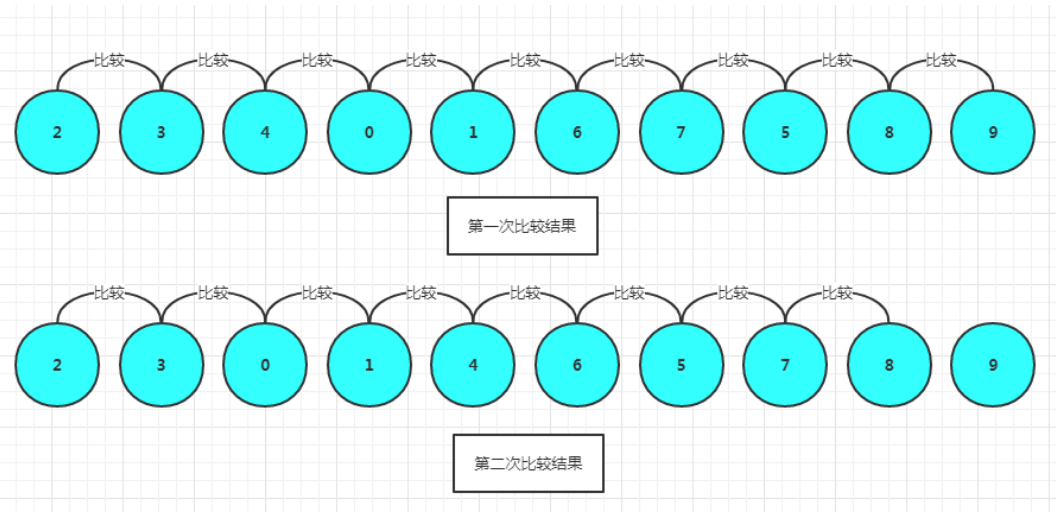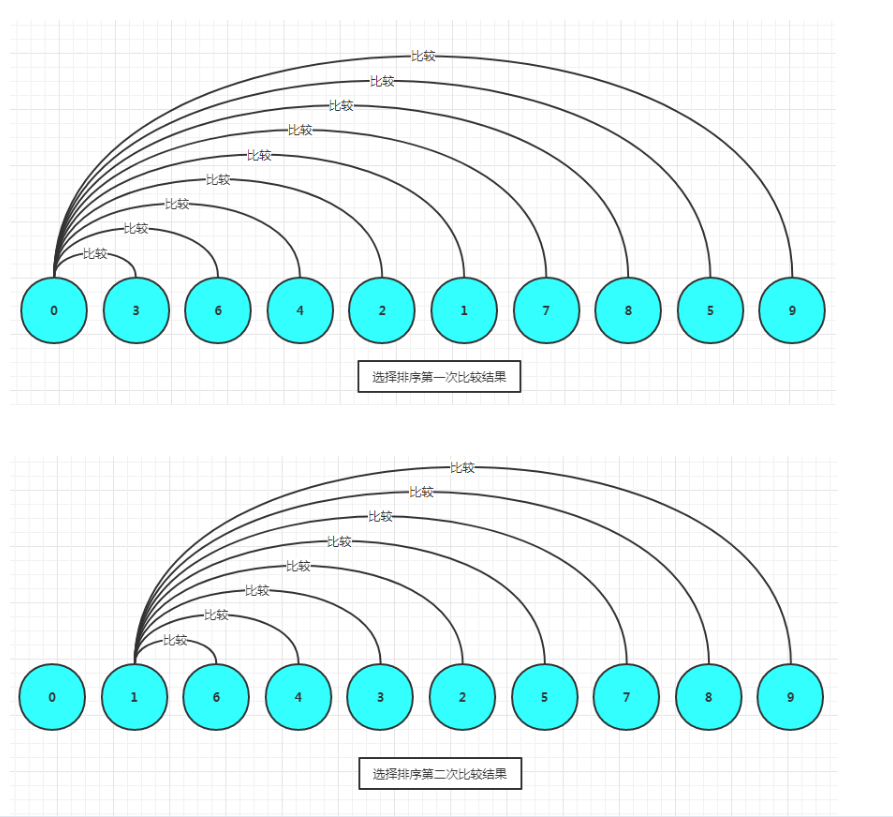# 冒泡排序原理

……冒泡排序算法：

 for (int j = 0; j < a.length - 1; j++) {
for (int i = 0; i < a.length - 1 - j; i++) {
if (a[i] > a[i + 1]) {
// change
temp = a[i + 1];
a[i + 1] = a[i];
a[i] = temp;
}
}
}

# 选择排序原理

……for (int j = 0; j <length- 1;j++) {
for (int i = j; i < length- 1; i++) {
if (a[j] > a[i + 1]) {
// change
temp = a[j];
a[j] = a[i + 1];
a[i + 1] = temp;
}
}
}

private static void seletSort(int[] a) {
int length = a.length;
int temp = 0;
int minIndex;
for (int j = 0; j <length- 1;j++) {
minIndex = j;
for (int i = j; i < length- 1; i++) {
if (a[minIndex] > a[i + 1]) {
minIndex = i+1;
}
}
temp = a[minIndex];
a[minIndex]= a[j];
a[j]=temp;
}
toString(a);
}

---------------------

posted @ 2019-02-22 15:41  绿色冰点  Views(8199)  Comments(0Edit  收藏